Teaching support from the UK’s largest provider of in-school maths tuition.one to one lessonsschools supported

Shape confident mathematicians

One to one tuition designed to build confidence and engagement in an interactive low-stakes environment

FREE secondary maths resources

Here you can find all of our free secondary resources; explore the collections or navigate to each section to filter resources by topicExplore all resources
Secondary Worksheets & Practice Questions

Shape confident mathematicians

One to one tuition designed to build confidence and engagement in an interactive low-stakes environment

# 15 Pythagoras Theorem Questions And Practice Problems (KS3 & KS4)

Pythagoras Theorem questions involve using the relationship between the sides of a right angled triangle to work out missing side lengths in triangles. Pythagoras Theorem is usually introduced towards the end of KS3 and is used to solve a variety of problems across KS4.

Here, you’ll find a selection of Pythagoras Theorem questions that demonstrate the different types of questions you are likely to encounter in KS3 and KS4, including several GCSE exam style questions.

### What is Pythagoras Theorem?

Pythagoras Theorem is the geometric theorem that states that the square of the hypotenuse (longest side) of a right angled triangle is equal to the sum of the squares of the two shorter sides of the triangle.

This can be written as a^2+b^2=c^2 for a triangle labelled like this:

### How to answer Pythagoras Theorem questions

1. Label the sides of the triangle a, b and c.
Note that the hypotenuse, the longest side of a right angled triangle, is opposite the right angle and will always be labelled \textbf{c}. The other two sides can be labelled a and b either way around.

2. Write down the formula and substitute the values.

a^2+b^2=c^2

You may be asked to give your answer in an exact form or round to a given degree of accuracy, such as a certain number of decimal places or significant figures.

### Pythagoras Theorem in real life

Pythagoras Theorem has many real life uses, including in architecture and construction, navigation and surveying.

### Pythagoras Theorem in KS3

Pythagoras Theorem is usually introduced towards the end of KS3.

The emphasis in KS3 is on students being able to:

• Correctly label a right angled triangle;
• Substitute values into the formula in order to work out the hypotenuse or one of the shorter sides.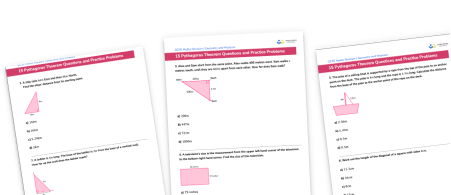15 Pythagoras Theorem Questions And Practice Problems WorksheetDownload FREE Now!

### Pythagoras Theorem KS3 questions

#### Non-calculator questions

1. A ship sails 6 \, km East and then 8 \, km North. Find the ship’s distance from its starting point.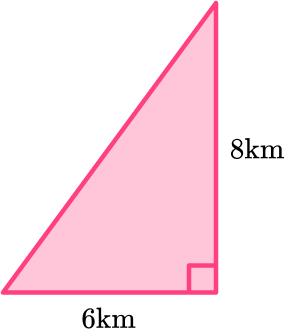14 \, km10 \, km5.29 \, km2 \, km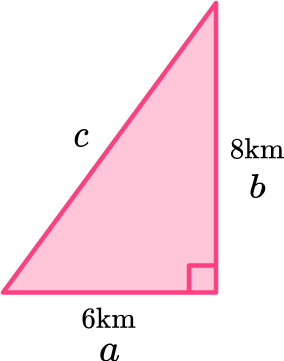\begin{aligned} a^2+b^2&=c^2\\ 6^2+8^2&=c^2\\ 36+64&=c^2\\ 100&=c^2\\ c&=\sqrt{100}\\ c&=10 \, km \end{aligned}

The ship is 10 kilometres from its starting point.

2. A ladder is 5 \, m long. The base of the ladder is 3 \, m from the base of a vertical wall. How far up the wall does the ladder reach?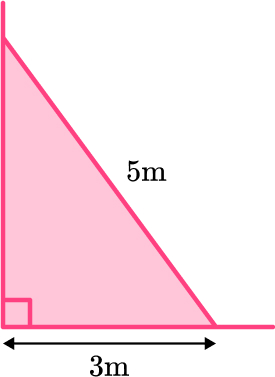8 \, m5.83 \, m4 \, m16 \, m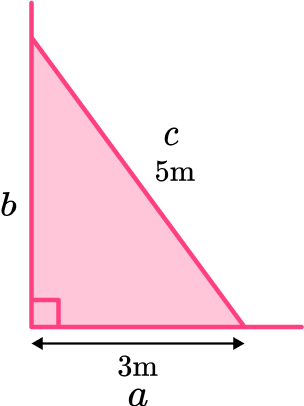\begin{aligned} b^{2}&=c^{2}-a^{2}\\ b^{2}&=5^{2}-3^{2}\\ b^{2}&=25-9\\ b^{2}&=16\\ b&=\sqrt{16}\\ b&=4 \, m \end{aligned}

The ladder reaches 4 meters up the wall.

#### Calculator questions

3. Alex and Sam start from the same point. Alex walks 400 metres west. Sam walks x metres south, until they are 600 \, m apart from each other. How far does Sam walk?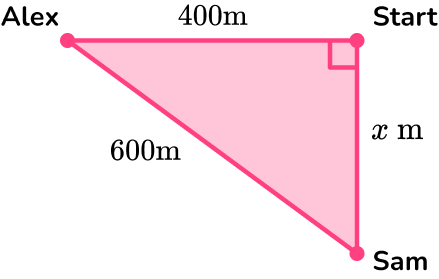200 \, m447 \, m721 \, m1000 \, m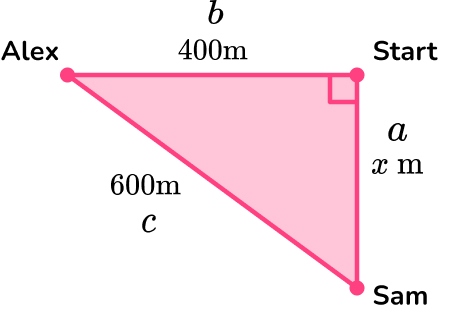\begin{aligned} a^{2}&=c^{2}-b^{2}\\ x^{2}&=600^{2}-400^{2}\\ x^{2}&=360000-160000\\ x^{2}&=200000\\ x&=\sqrt{200000}\\ x&=447.2135955\\ x&=447 \, m ~\text{(3sf)} \end{aligned}

4. A television’s size is the measurement from the upper left hand corner of the television to the bottom right hand corner. Find the size of this television.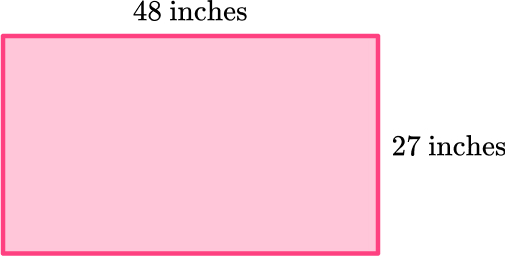75 inches150 inches39.7 inches55.1 inches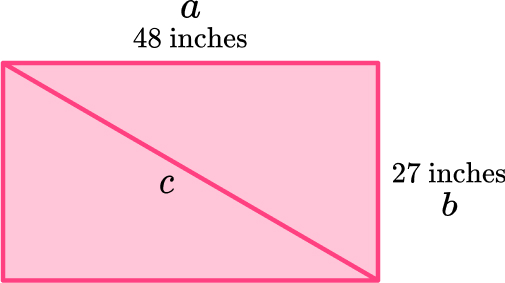\begin{aligned} a^2+b^2&=c^2\\ 48^2+27^2&=c^2\\ 2304+729&=c^2\\ 3033&=c^2\\ c&=\sqrt{3033}\\ c&=55.07267925\\ c&=55.1 \, \mathrm{inches} ~ \text{(3sf)} \end{aligned}

### Pythagoras Theorem in KS4

In KS4, students use Pythagoras Theorem to solve a variety of problems. Examples include:

• real life word problems
• coordinate problems
• multi-step problems
• 3D problems

Pythagoras Theorem may feature in questions alongside other topics, such as trigonometry, circle theorems or algebra.

The process for solving any Pythagoras Theorem problem always begins by identifying the relevant right angled triangle and labelling the sides a, b and c. If there is not a diagram in the question, it can be helpful to draw one.

#### Foundation GCSE Questions

5. The pole of a sailing boat is supported by a rope from the top of the pole to an anchor point on the deck. The pole is 4 \, m long and the rope is 4.5 \, m long. Calculate the distance from the base of the pole to the anchor point of the rope on the deck.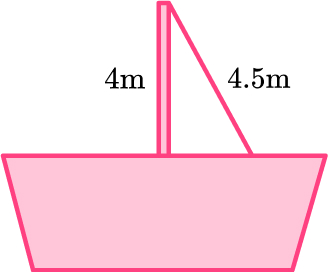2.06 \, m6.02 \, m8.5 \, m0.5 \, m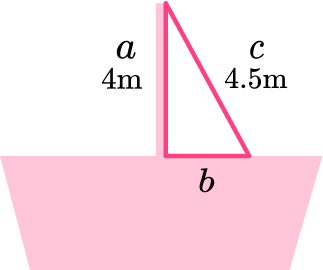\begin{aligned} b^{2}&=c^{2}-a^{2}\\ b^{2}&=4.5^{2}-4^{2}\\ b^{2}&=20.25-16\\ b^{2}&=4.25\\ b&=\sqrt{4.25}\\ b&=2.061552813\\ b&=2.06 \, m ~\text{(3sf)} \end{aligned}

6. Work out the length of the diagonal of a square with 8 \, cm sides.

11.3 \, cm16 \, cm8 \, cm12 \, cm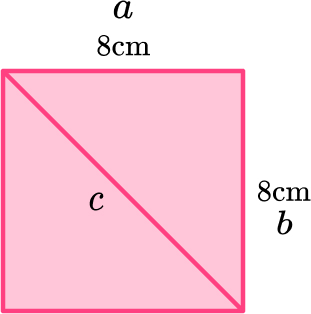\begin{aligned} a^2+b^2&=c^2\\ 8^2+8^2&=c^2\\ 64+64&=c^2\\ 128&=c^2\\ c&=\sqrt{128}\\ c&=11.3137085\\ c&=11.3 \, cm ~ \text{(3sf)} \end{aligned}

The diagonal of the square has a length of 11.3 centimetres.

7. ABC is an isosceles triangle.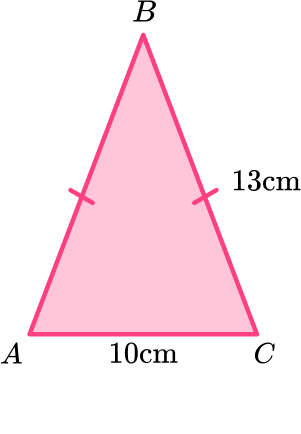Work out the height of the triangle.

8 \, cm12 \, cm8.31 \, cm16.4 \, cm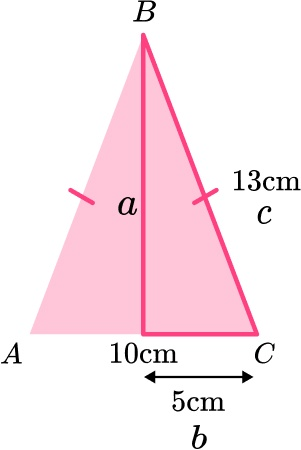\begin{aligned} a^{2}&=c^{2}-b^{2}\\ a^{2}&=13^{2}-5^{2}\\ a^{2}&=169-25\\ a^{2}&=144\\ a&=\sqrt{144}\\ a&=12 \, cm \end{aligned}

8. ABCD is an isosceles trapezium.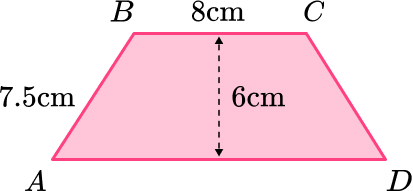Work out the length of AD.

4.5 \, cm12.5 \, cm17 \, cm23 \, cm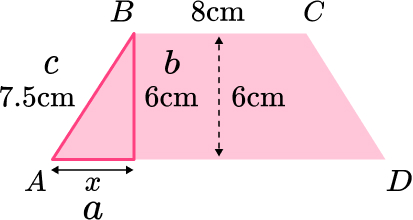\begin{aligned} a^{2}&=c^{2}-b^{2}\\ x^{2}&=7.5^{2}-6^{2}\\ x^{2}&=56.25-36\\ x^{2}&=20.25\\ x&=\sqrt{20.25}\\ x&=4.5 \, cm \end{aligned}

9. Here is a cm square grid. Calculate the distance between the points A and B.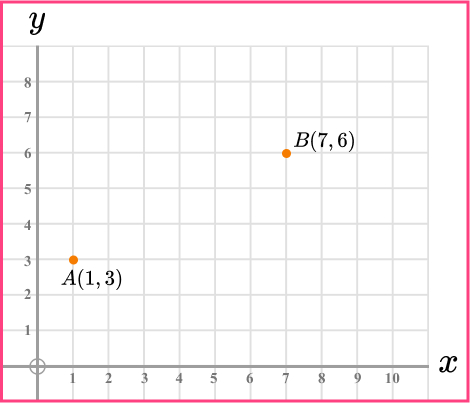9 \, cm5.20 \, cm3 \, cm6.71 \, cm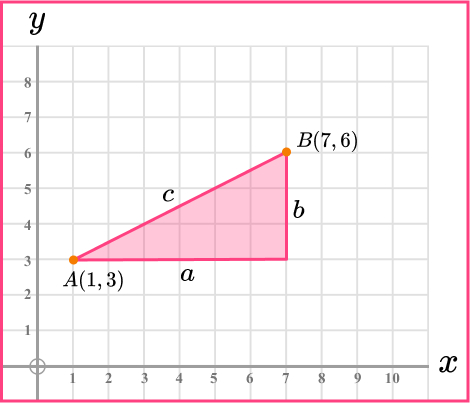\begin{aligned} a^2+b^2&=c^2\\ 6^2+3^2&=c^2\\ 36+9&=c^2\\ 45&=c^2\\ c&=\sqrt{45}\\ c&=6.708203932\\ c&=6.7 \, cm ~ \text{(3sf)} \end{aligned}

10. Which is a right angled triangle?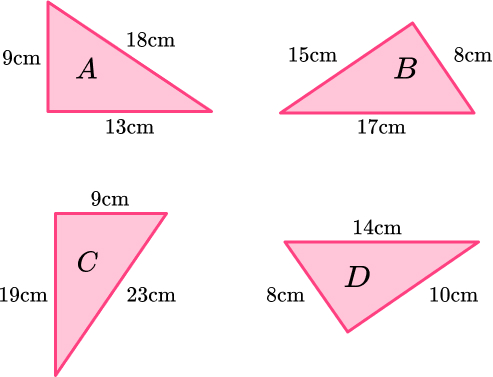ABCD\begin{aligned} \text{A: } 9^{2}+13^{2}&=250\\ 18^{2}&=324 \\ \end{aligned}

Not a right angled triangle because Pythagoras Theorem doesn’t work.

\begin{aligned} \text{B: } 8^{2}+15^{2}&=289\\ 17^{2}&=289 \\ \end{aligned}

Right angled triangle because Pythagoras Theorem works.

\begin{aligned} \text{C: } 9^{2}+19^{2}&=442\\ 23^{2}&=529 \\ \end{aligned}

Not a right angled triangle because Pythagoras Theorem doesn’t work.

\begin{aligned} \text{D: } 8^{2}+10^{2}&=164\\ 14^{2}&=196 \\ \end{aligned}

Not a right angled triangle because Pythagoras Theorem doesn’t work.

11. PQRS is made from two right angled triangles.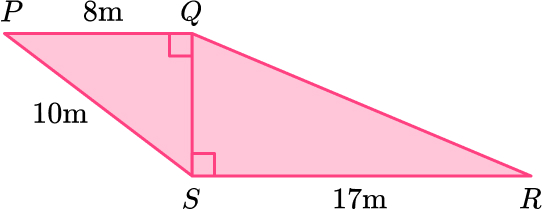Work out the length of QR.

18.0 \, m6 \, m15.9 \, m21.3 \, m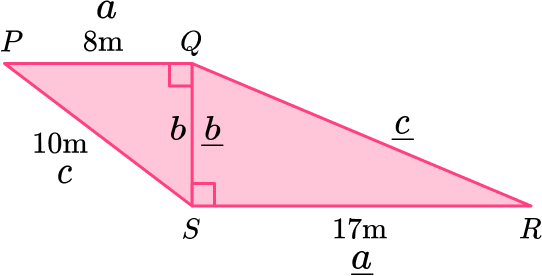Triangle \text{PQS:}

\begin{aligned} \\ b^{2}&=c^{2}-a^{2}\\ b^{2}&=10^{2}-8^{2}\\ b^{2}&=100-64\\ b^{2}&=36\\ b&=\sqrt{36}\\ b&=6 \, m \end{aligned}

Triangle \text{QRS}

\begin{aligned} \\ a^2+b^2&=c^2\\ 17^2+6^2&=c^2\\ 289+36&=c^2\\ 325&=c^2\\ c&=\sqrt{325}\\ c&=18.02775638\\ c&=18.0 \, m ~ \text{(3sf)} \end{aligned}

12. Here is a pattern made from right angled triangles. Work out the length x.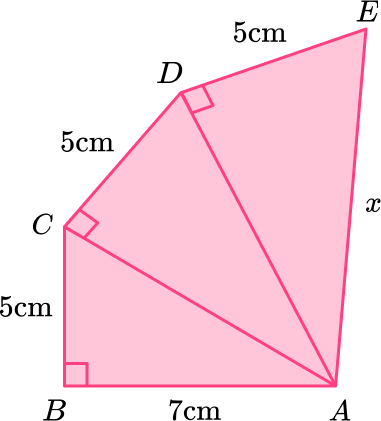8.60 \, cm16.6 \, cm11.1 \, cm8.66 \, cm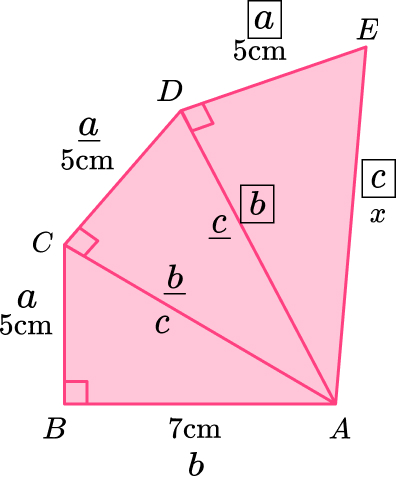Triangle \text{ABC:}

\begin{aligned} \\ a^2+b^2&=c^2\\ 5^2+7^2&=c^2\\ 74&=c^2\\ c&=\sqrt{74}\\ c&=8.602325267 \end{aligned}

Triangle \text{ACD:}

\begin{aligned} \\ a^2+b^2&=c^2\\ 5^2+8.60232567^2&=c^2\\ 99&=c^2\\ c&=\sqrt{99}\\ c&=9.949874371 \end{aligned}

\begin{aligned} \\ a^2+b^2&=c^2\\ 5^2+9.949874371^2&=c^2\\ 124&=c^2\\ c&=\sqrt{124}\\ c&=11.13552873\\ c&=11.1 \, cm ~ \text{(3sf)} \end{aligned}

### Higher GCSE Questions

13. Here is a pyramid.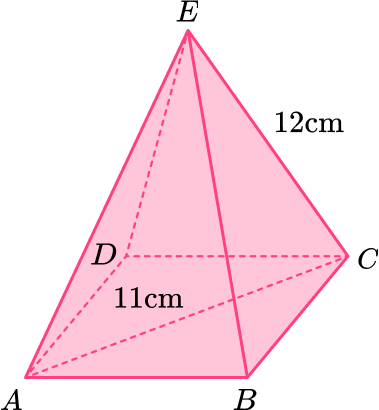Work out the height of the pyramid.

4.80 \, cm16.3 \, cm13.2 \, cm10.7 \, cm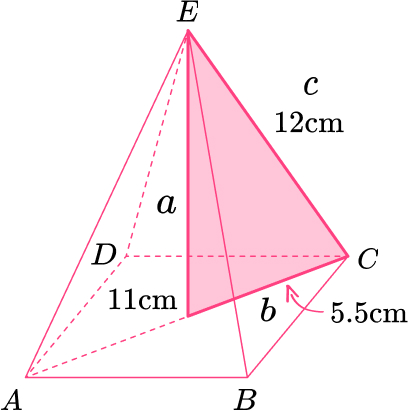\begin{aligned} a^{2}&=c^{2}-b^{2}\\ a^{2}&=12^{2}-5.5^{2}\\ a^{2}&=113.75\\ a&=\sqrt{113.75}\\ a&=10.6653645 \mathrm{cm} \\ a&=10.7 \, cm ~ \text{(3sf)} \end{aligned}

14. Here is a cuboid.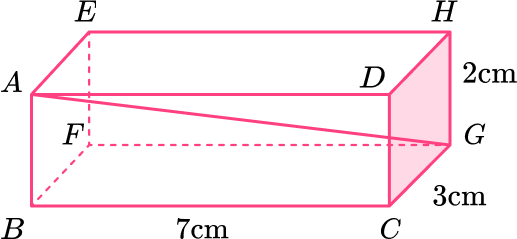Work out the length AG.

\sqrt{58} \, cm\sqrt{62} \, cm\sqrt{53} \, cm\sqrt{42} \, cm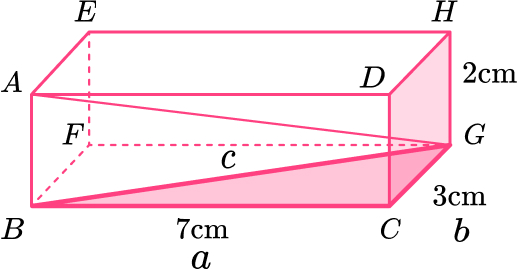Length of \text{BG:}

\begin{aligned} \\ a^2+b^2&=c^2\\ 7^2+3^2&=c^2\\ 58&=c^2\\ c&=\sqrt{58}\\ c&=7.615773106 \end{aligned}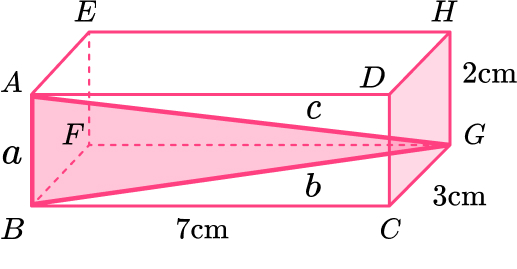Length of \text{AG:}

\begin{aligned} \\ a^2+b^2&=c^2\\ 2^2+7.615773106^2&=c^2\\ 62&=c^2\\ c&=\sqrt{62} \, cm \end{aligned}

15. Here is a right angled triangle.

Form an equation and use it to work out the value of x.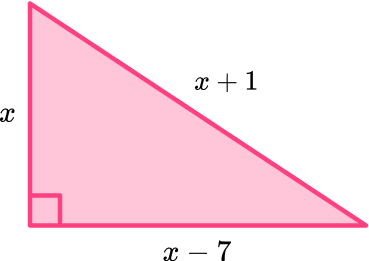4 \, cm10 \, cm17 \, cm12 \, cm\begin{aligned} x^{2}+(x-7)^{2}&=(x+1)^{2}\\ x^{2}+x^{2}-7x-7x+49&=x^{2}+x+x+1\\ 2x^{2}-14x+49&=x^{2}+2x+1\\ x^{2}-16x-48&=0\\ (x-4)(x-12)&=0 \end{aligned}

x=4 \, or \, x=12

x cannot be 4 as you cannot have a negative side length so x=12

How do you do Pythagoras questions?

Pythagoras Theorem is used to work out a missing length in a right angled triangle. If you have a right angled triangle and you know two of the lengths, label the sides of the triangle a, b and c ( c must be the hypotenuse – the longest side).

Pythagoras Theorem is a^2+b^2=c^2.

Substitute the values you know into Pythagoras Theorem and solve to find the missing side.

For a more detailed explanation, including a video and worked examples, see: Pythagoras Theorem.

How do you find the hypotenuse of a question?

The hypotenuse of a right angled triangle is the longest side. If you know the lengths of the other two sides, you can find the length of the hypotenuse by squaring the two shorter sides, adding those values together and then taking the square root.

By doing this you are finding c in a^2+b^2=c^2

How do you find the missing side of a triangle?

If your triangle is a right angled triangle and you know two of the sides, you can use Pythagoras Theorem to find the length of the third side. To do this, label the sides a, b and c (with c being the hypotenuse – the longest side). Substitute the values you know into a^2+b^2=c^2 and solve to find the missing side.

#### Looking for more Pythagoras Theorem questions and resources?

Third Space Learning’s free GCSE maths resource library contains detailed lessons with step-by-step instructions on how to solve Pythagoras Theorem problems, as well as maths worksheets with practice questions and more GCSE exam questions, based on past Edexcel, OCR and AQA exam questions.

Do you have students who need extra support in maths?
Every week Third Space Learning’s maths specialist tutors support thousands of students across hundreds of schools with weekly online 1-to-1 lessons and maths interventions designed to plug gaps and boost progress.

Since 2013 we’ve helped over 150,000 primary and secondary students become more confident, able mathematicians. Find out more about our GCSE Maths tuition or request a personalised quote for your school to speak to us about your school’s needs and how we can help.

Secondary school tuition designed to plug gaps and prepare KS3 and KS4 students for the challenges ahead.##### Beki Christian
Maths Teacher and Author
Beki has 11 years experience teaching secondary Maths. She is currently working as a Maths tutor as well as writing resources and blog posts for Third Space Learning.

Related Articles

x#### 15 Pythagoras Theorem Questions And Practice Problems Worksheet